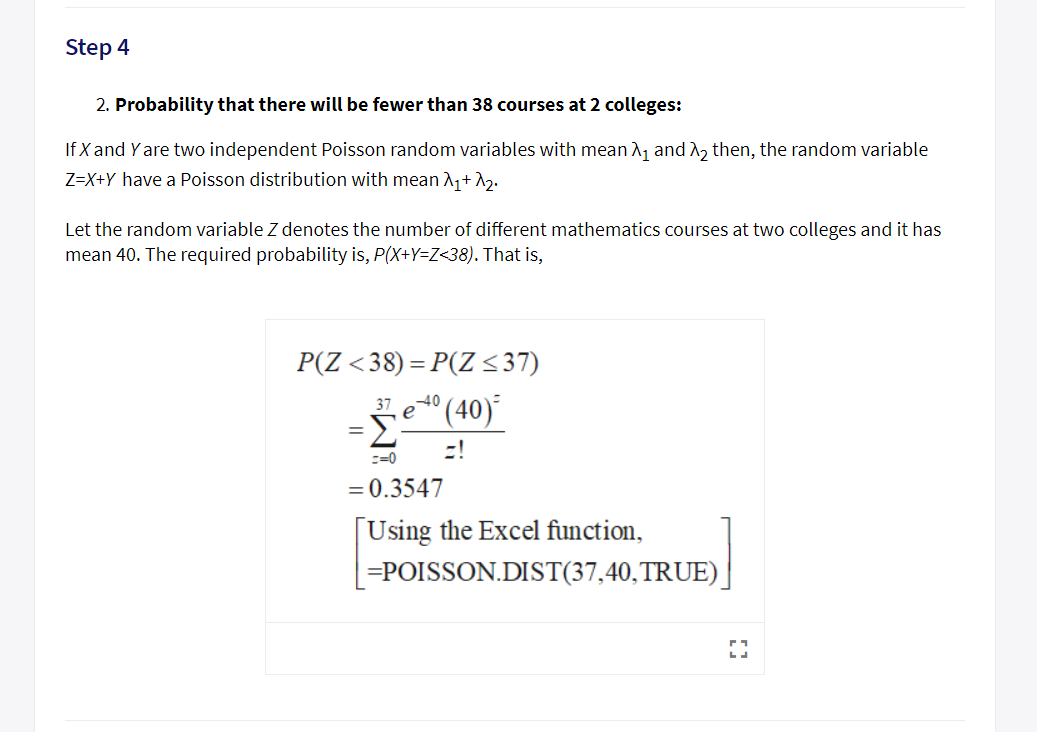Step 42. Probability that there wil be fewer than 38 courses at 2 colleges:If X and Y are two independent Poisson random variables with mean A1 and n2 then, the random variableZ-X+Y have a Poisson distribution with me2Let the random variable Z denotes the number of different mathematics courses at two colleges and it hasmean 40. The required probability is, P(XtY-Z-38). That is,3740C-00.3547Using the Excel function,POISSON.DIST(37,40,TRUE)

Question

The number of different mathematics courses at community colleges has a Poisson Distribution with a mean of 20 courses per college.

*show your basic keystrokes and round your answer to 4 decimal places.

1. What is the probability there will be fewer than 38 courses at 2 colleges?

Someone on this site already answered this question, but I don't understand what they did, how they got the number 40 for this problem to solve it. Can you help me understand this please? I also didn't know if this was poissoncdf or poissonpdf.help_outlineImage TranscriptioncloseStep 4 2. Probability that there wil be fewer than 38 courses at 2 colleges: If X and Y are two independent Poisson random variables with mean A1 and n2 then, the random variable Z-X+Y have a Poisson distribution with me2 Let the random variable Z denotes the number of different mathematics courses at two colleges and it has mean 40. The required probability is, P(XtY-Z-38). That is, 3740 C-0 0.3547 Using the Excel function, POISSON.DIST(37,40,TRUE) fullscreen
Step 1

The Poisson distribution:

A discrete random variable X is said to have a Poisson distribution with parameter λ then the probability mass function (pmf) of the random variable is given by:

Step 2

Additive property of two independent Poisson random variable:

If two independent random variables X1 and X2 follow a Poisson distribution with parameter(mean) λ1 and λ2 respectively then their sum also follow a Poisson distribution with parameter λ1 + λ2. That is, X follows P (λ1) and Y follows P (λ2) then X1+ X2 follows P (λ1 + λ2).

Step 3
1. Calculation:

The number of different mathematics courses at community college has a Poisson distribution with mean 20 courses per college. For Poisson distribution mean is same as the parameter. The random variable   X1 denote number of different mathematics courses at first community college. The random variable   X2 denote number of different mathematics courses at second community college. It is known that   number of different mathematics courses at community college has a Poisson distribution with mean (parameter) 20 courses per college. The random variables X1 and X2 are independent also. Therefore, each random variable X1 and X2 follows Poisson distri...

Want to see the full answer?

See Solution

Want to see this answer and more?

Our solutions are written by experts, many with advanced degrees, and available 24/7

See Solution
Tagged in

Statistics